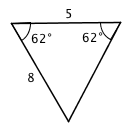Home > GC > Chapter 6 > Lesson 6.2.2 > Problem6-51

6-51.
1. Determine if each pair of triangles below are congruent, similar but not congruent, or if they are neither. If they are congruent, organize your reasoning into a flowchart. Homework Help ✎

1.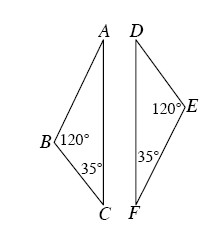2.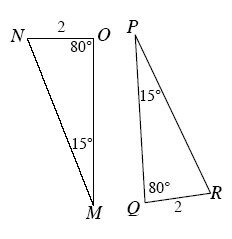3.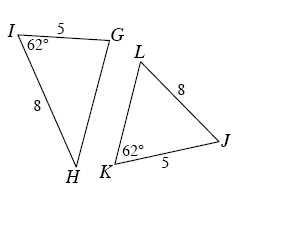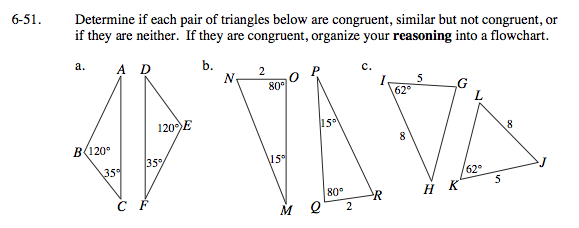Use the triangle congruence shortcuts explained in the Math Notes box in Lesson 6.1.3.

Similar by AA~.

ΔMON ≅ ΔPQR

AAS ≅ or ASA ≅

For the two triangles to be congruent, they should look like the diagram shown below. Is this triangle possible? If the triangles are not congruent, is it possible for them to be similar?